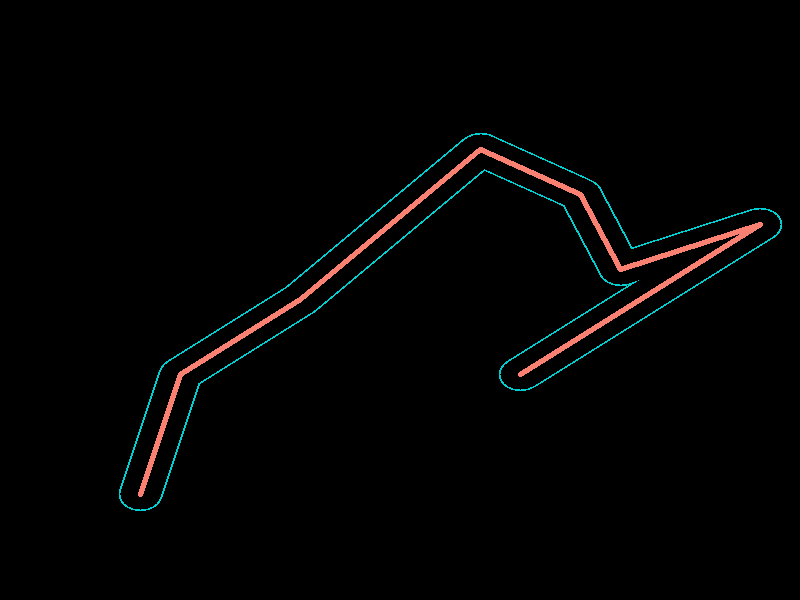# Proximity#

The Proximity tools allow you to perform analysis that accounts for one of three distance metrics which are Euclidean (straight-line), Mahattan, and Great Cricle.

Proximity Distance: Calculates distance to the nearest source for each pixel in the input raster.

Proximity Allocation: Identifies the nearest source pixel (allocation) for each cell in the input raster.

Proximity Direction: Calculate direction to the nearest source for each cell in the input raster.

## Importing Packages#

:

import numpy as np

import xrspatial


## Proximity Distance#

The xrspatial.proximity function operates on a given aggregate to produce a new distance aggregate based on target values and a distance metric. The values in the new aggregate will be the distance (according to the given metric) between each array cell (pixel) and the nearest target value in the source aggregate.

A powerful feature of proximity is that you can target specific values, the target_values parameter, in the aggregate for distance calculation, while others are ignored. If it is not set, then the default targets will be all non-zero pixels. Play with the parameter and see the difference of using target_values=[1,2,3,4] vs. default option.

### Load data and create ds.Canvas#

:

from xrspatial import proximity
import pandas as pd

df = pd.DataFrame({
'x': [-13, -11, -5, 4, 9, 11, 18, 6],
'y': [-13, -5, 0, 10, 7, 2, 5, -5],
'id': [1, 2, 3, 4, 5, 6, 7, 8]
})

W = 800
H = 600

cvs = ds.Canvas(plot_width=W, plot_height=H,
x_range=(-20, 20), y_range=(-20, 20))


### Create Proximity Aggregate#

• Use Canvas.points to create an xarray.DataArray that all non-zero cells have an unique value that represent for a separate region/source.

• Calculate proximity to nearest target using xrspatial.proximity

:

# all non-zero cells have an unique value that represent for a separate region
points_agg = cvs.points(df, x='x', y='y', agg=ds.min('id'))
points_agg.data[~np.isfinite(points_agg.data)] = 0
points_agg = (points_agg).astype(int)

threshold=1,
max_px=5)

:### Create proximity grid for all a specific set of target values#

:

targets = [1, 2, 3, 4]
target_proximity_agg = proximity(points_agg, target_values=targets, distance_metric="GREAT_CIRCLE")


:### Create proximity grid for all non-zero values#

:

proximity_agg = proximity(points_agg, distance_metric="GREAT_CIRCLE")


::

line_agg = cvs.line(df, x='x', y='y')
threshold=1,
max_px=2)

::

line_proximity = proximity(line_agg)

:### Transform Proximity DataArray#

Like the other Datashader spatial tools, the result of proximity is an xarray.DataArray with a large API of potential transformations.

Below is an example of using DataArray.where() to apply a minimum distance and maximum distance.

:

where_clause = (line_proximity > 1) & (line_proximity < 1.1)

:## Proximity Allocation#

Similar to xrspatial.proximity, the xrspatial.allocation function operates on a given aggregate to produce the nearest source (instead of distance to nearest source) based on target values and a distance metric. The values in the new aggregate will be the value of the nearest feature.

In the same way, you can target specific values with the target_values parameter.

:

from xrspatial import allocation

allocation_agg = allocation(points_agg, distance_metric="GREAT_CIRCLE")


:## Proximity Direction#

Similar to xrspatial.proximity, the xrspatial.direction function operates on a given aggregate. It calculates for each pixel the direction to nearest source (instead of distance) based on target values and a distance metric. The output values are based on compass directions, range from 0 to 360: - 0 is for the source cell itself - 90 is the east - 180 is the south - 270 is the west - 360 is the north

In the same way, you can target specific values with the target_values parameter.

:

from xrspatial import direction

direction_agg = direction(points_agg, distance_metric="GREAT_CIRCLE")


: